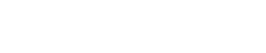# BEHAVIOUR OF TENSION MEMBERS UNDER LOADSReading time: 1 minute

Behaviour of tension members under the action of loads for tension ties and is discussed below:

#### Behaviour of Tension Tie:

The tie is the simplest type of member in tension. When a tensile load is applied on one side of a tie fixed at one end as shown in figure below, the applied load causes reaction at one end and the extension of members will take place.Tensile stress and tensile strain is then calculated for the members as below:

Tensile Strain = (Tensile Stress/Youngs Modulus)

#### Behaviour of Cable members under Loads:Cables have tensile strength but have negligible resistance to bending moments and shear. Cables hangs in catenary due to self-weight as shown in figure above.

Cables when subjected to a point load (P) the working line would change shape as shown since it can only act in pure tension.The figures with two point loads, uniformly distributed load are shown here. It can be seen that tensile structures of this type deflect to a profile which is same shape as the equivalent bending moment diagram.